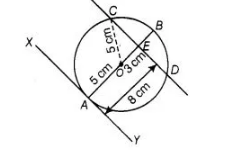# At one end A of a diameter AB

Question:

At one end A of a diameter AB of a circle of radius 5 cm, tangent XAY is drawn to the circle. The length of the chord CD parallel to XY and at a

distance 8 cm from A, is

(a) 4 cm

(b) 5 cm

(c) 6 cm

(d) 8 cm

Solution:

(d) First, draw a circle of radius 5 cm having centre 0. A tangent XY is drawn at point A.A chord $C D$ is drawn which is parallel to $X Y$ and at a distance of $8 \mathrm{~cm}$ from $A$.

Now, $\angle O A Y=90^{\circ}$

[Tangent and any point of a circle is perpendicular to the radius through the point of contact]

$\triangle O A Y+\triangle O E D=180^{\circ}$ $\left[\because\right.$ sum of cointerior is $\left.180^{\circ}\right]$

$\Rightarrow$ $\triangle O E D=180^{\circ}$

Also, $A E=8 \mathrm{~cm} .$ Join $O C$

Now, in right angled $\triangle O E C$, $O C^{2}=O E^{2}+E C^{2}$

$\Rightarrow$ $E C^{2}=O C^{2}-O E^{2}$ [by Pythagoras theorem]

$=5^{2}-3^{2}$

$[\because O C=$ radius $=5 \mathrm{~cm}, O E=A E-A O=8-5=3 \mathrm{~cm}]$

$=25-9=16$

$\Rightarrow \quad E C=4 \mathrm{~cm}$

Hence, length of chord $C D=2 C E=2 \times 4=8 \mathrm{~cm}$

[since, perpendicular from centre to the chord bisects the chord]